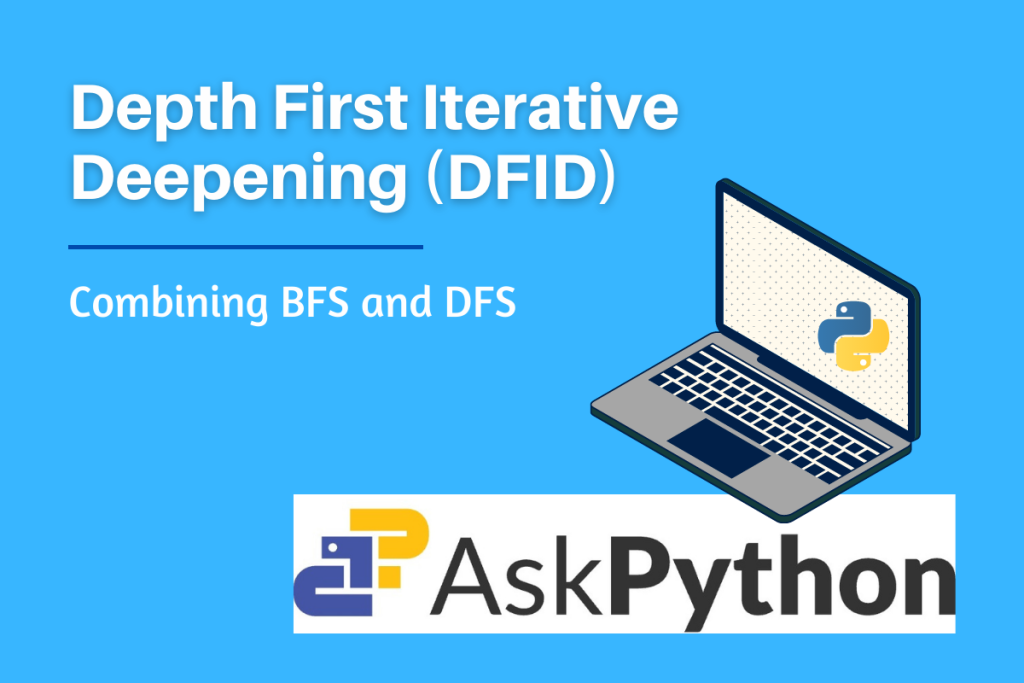# Depth First Iterative Deepening (DFID) Algorithm in PythonHello readers, this article let us understand what exactly is Depth First Iterative Deepening (DFID) also known as Iterative Deepening Search (IDS). Its advantages, applications, and implementation in python.

## What is Depth First Iterative Deepening Search?

Depth First Iterative Deepening is an iterative searching technique that combines the advantages of both Depth-First search (DFS) and Breadth-First Search (BFS).

While searching a particular node in a graph representation Breadth-First Search requires lots of space thus increasing the space complexity and the Depth-First search takes a little more time thus this search strategy has much time complexity and also Depth-First search does not always find the cheapest path. To overcome all these drawbacks of Depth-First search and Breadth-First Search, Depth First Iterative Deepening Search is implemented.

## How does DFIDS work?

DFID expands all nodes at a given depth before expanding any nodes at greater depth.So it is guaranteed to find the shortest path or optimal solution from start to goal state. The working of the DFID algorithm is shown in Figure

At any given time, it is performing a DFS and never searches deeper than depth ‘d’. Thus, the space it uses is O(d). The disadvantage of DFID is that it performs wasted compotation before reaching the goal depth.

## DFID Algorithm

• INPUT: START and GOAL states
• LOCAL VARIABLE: Found
• METHOD
• Initialise d = 1 and FOUND = False
• while (FOUND = False) do
• perform DFS from start to depth d.
• if goal state is obtained then FOUND = True else discard the nodes generated in the search of depth d.
• d = d + 1
• if FOUND = true, then return the depth.
• Stop

## Implementation of Depth First Iterative Deepening in Python

Implementing graph

```class Node:
def __init__(self, val=None):
self.val = val
self.left = None
self.right = None

def get_root():
values = iter([3, 8, 6, 9, None, None, 11, 10, None, None,
12, None, None, 7, None, None, 4, 5, None, None, 13, None, None])

def tree_recur(itr):
val = next(itr)
if val is not None:
node = Node(val)
node.left = tree_recur(itr)
node.right = tree_recur(itr)
return node

return tree_recur(values)
```

Function for DFIDS

```def dfids():
root = get_root()
res = float("inf")

def dfids_search(node, depth, limit):
if depth <= limit and node is not None:
val = node.val
if val == 12:
nonlocal res
res = min(res, depth)
else:
dfids_search(node.left, depth + 1, limit)
dfids_search(node.right, depth + 1, limit)

for limit in range(1,5):
dfids_search(root, 0, limit)
if res < float("inf"):
return res
return -1

if __name__ == "__main__":
print("\nShortest Depth: ", dfids())
```

## Applications of Depth First Iterative Deepening

Depth First Iterative Deepening Search is used to find optimal solutions or the best-suited path for a given problem statement. It is preferred to use this search strategy when a large state space is provided and no information on the depth of solution is mentioned. Following are a few applications of DFIDS

• Artificial Intelligence and Data Science- analyzing network
• Puzzle-solving with a unique solution (example: sudoku)
• Detecting cycle in a graph.
• Sorting Directed Acyclic Graph (DAG)
• N- Queens problem

## Summary

In this article, we have thoroughly studied Depth First Iterative Deepening Search, its importance and its implementation.